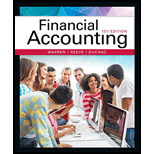# Ware Co. produces and sells motorcycle parts. On the first day of its fiscal year, Ware Co. issued $35,000,000 of five-year, 12% bonds at a market (effective) interest rate of 10%, with interest payable semiannually. Compute the following, presenting figures used in your computations: a. The amount of cash proceeds from the sale of the bonds. Use the tables of present values in Exhibits 8 and 10. Round to the nearest dollar. b. The amount of premium to be amortized for the first semiannual interest payment period, using the interest method. Round to the nearest dollar. c. The amount of premium to be amortized for the second semiannual interest payment period, using the interest method. Round to the nearest dollar. d. The amount of the bond interest expense for the first year.BuyFindarrow_forward ### Financial Accounting 15th Edition Carl Warren + 2 others Publisher: Cengage Learning ISBN: 9781337272124 #### Solutions Chapter SectionBuyFindarrow_forward ### Financial Accounting 15th Edition Carl Warren + 2 others Publisher: Cengage Learning ISBN: 9781337272124 Chapter 14, Problem 25E Textbook Problem 157 views ## Ware Co. produces and sells motorcycle parts. On the first day of its fiscal year, Ware Co. issued$35,000,000 of five-year, 12% bonds at a market (effective) interest rate of 10%, with interest payable semiannually. Compute the following, presenting figures used in your computations:        a.       The amount of cash proceeds from the sale of the bonds. Use the tables of present values in Exhibits 8 and 10. Round to the nearest dollar.       b.       The amount of premium to be amortized for the first semiannual interest payment period, using the interest method. Round to the nearest dollar.        c.       The amount of premium to be amortized for the second semiannual interest payment period, using the interest method. Round to the nearest dollar.       d.       The amount of the bond interest expense for the first year.

(a)

To determine

Calculate the amount of cash proceeds (present value) from the sale of the bonds.

### Explanation of Solution

Bonds Payable: Bonds payable are referred to long-term debts of the business, issued to various lenders known as bondholders, generally in multiples of $1,000 per bond, to raise fund for financing the operations. Premium on bonds payable: It occurs when the bonds are issued at a high price than the face value. Effective-interest method of amortization: It is an amortization model that apportions the amount of bond discount or premium based on the market interest rate. Present Value: The value of today’s amount expected to be paid or received in the future at a compound interest rate is called as present value. Determine the amount of cash proceeds (present value) from the sale of the bonds. Step 1: Calculate the semiannual interest on bonds. Interest=Face value×Face interest rate×Interest time period=$35,000,000×12%×612=$2,100,000 Step 2: Calculate the present value of interest.  Particulars Amount Interest payment (a)$2,100,000 PV factor at semiannual market interest rate of 5% for 10 periods (b) 7.72173 Present value (a)×(b) \$16,215,633

Table (1)

Note: The present value factor for 10 periods at 5% interest would be 7

(b)

To determine

Calculate the amount of premium to be amortized for the first semiannual interest payment period.

(c)

To determine

Calculate the amount of premium to be amortized for the second semiannual interest payment period.

(d)

To determine

Calculate the amount of bond interest expense for first year.

### Still sussing out bartleby?

Check out a sample textbook solution.

See a sample solution

#### The Solution to Your Study Problems

Bartleby provides explanations to thousands of textbook problems written by our experts, many with advanced degrees!

Get Started